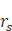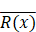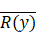# 斯皮尔曼相关系数(Spearman correlation coefficient)介绍及其计算例

1. 什么是秩相关系数(Correlation coefficient)？

2. 单调性，monotonicity

3. 斯皮尔曼秩相关系数(Correlation coefficient)

4. 什么时候使用斯皮尔曼秩相关系数(Correlation coefficient)呢？

5. 斯皮尔曼秩相关系数(Correlation coefficient)计算公式

6. 斯皮尔曼秩相关系数(Correlation coefficient)计算例

6.1 手动计算

6.2 scipy函数

6.3 pandas corr()

# 1. 什么是秩相关系数(Correlation coefficient)？

秩相关系数(Correlation coefficient)Coefficient of Rank Correlation），又称等级相关系数(Correlation coefficient)，反映的是两个随机变量(random variable)的的变化趋势方向和强度之间的关联，是将两个随机变量(random variable)的样本值按数据的大小顺序排列位次，以各要素样本值的位次代替实际数据而求得的一种统计量它是反映等级相关程度的统计分析指标，常用的等级相关分析方法有Spearman相关系数(Correlation coefficient)Kendall秩相关系数(Correlation coefficient)等。主要用于数据分析。

这里的秩是啥意思呢？我第一次看到这个词的时候第一感是它跟矩阵(matrix)的秩(Rank)有啥关系，没有关系。这里是秩序的秩，或者说排名、顺序、等级的意思(写成ranked或者ranking的话就不容易误解了)。

考虑两个随机变量(random variable)XY，如果秩相关系数(Correlation coefficient)为正，则随着X的增加而增加；如果秩相关系数(Correlation coefficient)为负，则Y随着X的增加而减小；如果秩相关系数(Correlation coefficient)为0，则表示随着Y的增减变化跟X的增减变化没啥关系。当YX越来越接近严格单调的函数关系时，秩相关系数(Correlation coefficient)在数值上就越来越大。当秩相关系数(Correlation coefficient)为1或者-1时，就表明Y随着X的增加而严格单调增加或单调减小。

在实际应用中，有时获得的原始资料没有具体的数据表现，只能用等级来描述某种现象，要分析现象之间的相关关系，就只能用秩相关系数(Correlation coefficient)。

# 2. 单调性，monotonicity

为了理解斯皮尔曼相关系数(Correlation coefficient)，首先需要了解什么是单调性和单调函数。一个单调函数是指随着它的自变量(independent variable)增大，函数值(因变量)要么总是增大（单调递增）要么总是变小（单调递减），而不会有时变大、有时变小（不是单调函数）。

如上图所示，左图代表的是一个单调递增函数，中间图代表的是一个单调递减函数，而右边的图所代表的函数则不是单调函数。

# 3. 斯皮尔曼秩相关系数(Correlation coefficient)

斯皮尔曼秩相关系数(Correlation coefficient)(The Spearman’s rank coefficient of correlation)，简称斯皮尔曼相关系数(Correlation coefficient)，是秩相关(rank correlation)的一种非参数(non-parametric)度量(nonparametric measure)。得名于英国统计学家Charles Spearman，通常记为希腊字母‘ρ’ (rho)( often called Spearman's rho)或者在讨论斯皮尔曼相关系数(Correlation coefficient)之前，首先要理解皮尔逊相关(Pearson’s correlation)，斯皮尔曼相关可以看作是皮尔逊相关的非参数(non-parametric)版本（nonparametric version）。皮尔逊相关是关于两个随机变量(random variable)之间的线性关系强度的统计度量(statistical measure)，而斯皮尔曼相关考察的是两者单调关系（monotonic relationship）的强度，通俗地说就是两者在变大或变小的趋势上多大程度上保持步调一致，哪怕没有保持比例关系。计算皮尔逊相关系数(Correlation coefficient)时使用的是数据样本值本身，而计算斯皮尔曼相关系数(Correlation coefficient)使用的是数据样本排位位次值（有时候数据本身就是位次值，有时候数据本身不是位次值，则在计算斯皮尔曼相关系数(Correlation coefficient)之前要先计算位次值）。

# 4. 什么时候使用斯皮尔曼秩相关系数(Correlation coefficient)呢？

能够适用皮尔逊相关的场合当然是优先使用皮尔逊相关，但是在有些场合，皮尔逊相关所需要的前提假设(Hypothesis)不能得到满足，这是就可以考虑使用斯皮尔曼相关，比如说以下一些情况下：

1. 如果你的数据展现的是非线性关系，或者不是正态分布(normal distribution)(Distribution)的。
2. 如果至少有一方数据是序数类型（ordinal）而非数值类型。比如说，如果数据的赋值为"第一、第二、第三、… "你就是在处理序数类型数据。更具具体一点的例子就是，比如说你考察两个球队在历年联赛中的战绩之间的关系，那么你得到的数据可能是这样的：A队在2010~2020年间的联赛排名为{1,2,4,5,…,2}, B队在2010~2020年间的联赛排名为{2,1,3,6,…,4}。这两个数据就是序数类型的数据，考察它们的相关性你使用皮尔逊相关系数(Correlation coefficient)就不妥当
3. 如果数据中有明显的异常值（outliers）。与皮尔逊相关不同，斯皮尔曼相关对于异常值不太敏感，因为它基于排序位次进行计算，实际数值之间的差异大小对于计算结果没有直接影响

1. 受教育水平更高的人更关心环境吗？
2. 患者的症状数与他们服药的意愿有关系吗？
3. 球队的联赛成绩（名次）与他们所在城市的经济发展水平有关系吗？

# 5. 斯皮尔曼秩相关系数(Correlation coefficient)计算公式

取决于观测数据中有没有位次相同的数据(the same rank assigned to two or more observations),斯皮尔曼相关系数(Correlation coefficient)可以以以下两种方法之一进行计算。

当没有位次相同的数据时，可以用一个更简单的公式，如下所示：

其中:

• di 表示第i个数据对的位次值之差
• n 总的观测样本数

如果观测样本中存在位次相同的数据，则需要使用如下所示的全版本的计算公式：

• R(x) 和 R(y) 分别是x和y的位次
•和分别表示平均位次

# 6. 斯皮尔曼秩相关系数(Correlation coefficient)计算例

在很多软件工具或者工具库里都有各种相关系数(Correlation coefficient)的计算。在以下Ref1中介绍了在Excel中如何计算斯皮尔曼相关系数(Correlation coefficient)，有兴趣的小伙伴可以参考。

## 6.1 手动计算

在Ref2中给出了一个手动计算例（最低限度的手动计算对于掌握一个新的概念是绝对必要的，手动计算能帮助更加深刻地理解本质），如下表所示：

这是9个学生的历史和地理的成绩和排名，每一行从左到右分别历史科目的分数、历史成绩中的排名、地理科目的分数、地理成绩中的排名，排名位次之差、排名位次的平方。由于数据中没有位次相同的数据，所以可以用简单版本的公式进行计算，如下所示：

这个结果意味着这9个学生历史成绩排名和地理成绩呈现出很强的单调关系，即历史成绩好非常大概率地理成绩也非常好，反之依然。其实目测上表也基本上可以得出这个印象。只不过斯皮尔曼相关系数(Correlation coefficient)把这种印象进行精确的量化。

## 6.2 scipy函数

以下介绍scipy中计算斯皮尔曼相关系数(Correlation coefficient)的函数scipy.stats.spearmanr的使用例。

scipy.stats.spearmanr(ab=Noneaxis=0nan_policy='propagate'alternative='two-sided')

函数调用接口请参阅scipy文档，这里只介绍一些要点。

该函数返回的结果除了相关系数(Correlation coefficient)外，还包含一个p值，大致来说就是表示由完全不相关系统所生成的数据能够计算得出跟当前待测数据对所计算结果相当的斯皮尔曼相关系数(Correlation coefficient)的概率有多大。P值不是完全可靠的，但是对于大于500的数据集(Dataset)其估计结果基本还是合理的。

计算例1：调用scipy函数计算以上数据的斯皮尔曼相关系数(Correlation coefficient)。

``````import numpy as np
from scipy import stats
stats.spearmanr([3,5,1,6,7,2,8,9,4], [5,3,2,6,8,1,7,9,4])``````

结果：SpearmanrResult(correlation=0.9, pvalue=0.0009430623223403293)

得到了跟手动计算相同的结果，安心ing^-^。

另外p-value仅为0.0009，这说明历史成绩和地理成绩几乎不可能是不相关，反过来说就是它们是非常相关的。

计算例2：两个随机数序列的斯皮尔曼相关系数(Correlation coefficient)

``````rng = np.random.default_rng()
x2n = rng.standard_normal((100, 2))
stats.spearmanr(x2n)``````

结果：SpearmanrResult(correlation=-0.00876087608760876, pvalue=0.931061841564777)

嗯，相关度极低。。。诚不欺我^-^

## 6.3 pandas corr()

Pandas也提供了计算斯皮尔曼相关系数(Correlation coefficient)的函数，事实上是提供了一个通用(GPU general purpose GPU)的相关系数(Correlation coefficient)计算函数接口，通过method参数可以指定是需要计算那种相关系数(Correlation coefficient)。如下例所示：

``````import pandas as pd
import numpy as np
X=pd.Series([3,5,1,6,7,2,8,9,4])
Y=pd.Series([5,3,2,6,8,1,7,9,4])
rho = X.corr(Y,method='spearman')
print(rho)
``````

同样得到结果为0.9，只不过没有像scipy函数那样提供一个p-value。

[Reference]

 https://docs.scipy.org/doc/scipy/reference/generated/scipy.stats.spearmanr.html

(0)